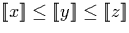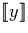Next: Limits within [-1,1] Up: High-Level Operations Previous: High-Level Operations

# Computing Limits

In order to compute limits, we first define a function which takes three signed binary streams (x,y,z) as inputs with the property. The function examines the streams x and z and generates a (possibly empty) string of digits whose range (see section 3.1) contains all possible values for y and then uses the stream y directly to return the remainder of the output such that the whole output stream represents the number. Importantly, this function determines information about y from the bounds x and z before examining y itself.

In order to obtain an algorithm for computing limits, we require that this function has the property that if x and z are closer than some finite amount a digit is generated, and that if x and z are equal, y is not examined at all.

The function may be used recursively to convert a real represented as a stream of nested intervals (section 3.5) into a single signed binary stream by trying to find common digits from the first interval in the stream of nested intervals when possible, and then using the remaining intervals to determine the rest of the signed binary stream. When we have determined digits from the first interval (or determined that they end-points of the stream are not equal), we examine the next intervals. We can use the `p' function described in section 4.1.4 to re-express the endpoints of the next interval starting with the digits already generated, and then remove these known digits from the endpoints. This allows us to then use the original function again to determine the further digits in the output stream.

We know that because the nested intervals converge to a single value, we can get arbitrarily many output digits by examining a finite number of intervals.

The motivation for defining such a function is that now, in order to compute the limit of a given Cauchy sequence, we can express the sequence as a stream of nested lower and upper bounds on the limit and then simply convert this into a signed binary stream as described. Many useful functions can be defined as the limits of Cauchy sequences.Next: Limits within [-1,1] Up: High-Level Operations Previous: High-Level Operations
Martin Escardo
5/11/2000